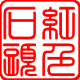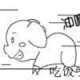﻿ 读者墙 – 互联网新鲜事

•9+
•6+
•5+
•5+
•4+
•3+
•3+
•3+
•3+
•2+
•2+
•2+
•2+
•2+
•2+
•1+
•1+
•1+
•1+
•1+
•1+
•1+
•1+
•1+
•1+
•1+
•1+
•1+
•1+
•1+
•1+
•1+
•1+
•1+
•1+
•1+
•1+
•1+
•1+
•1+
•1+
•1+
•1+
•1+
•1+
•1+
•1+
•1+
•1+
•1+
•1+